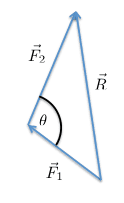# Force Resultants: Comparing Rules

• Bassel AbdulSabour
I agree. I am trying to interpret what the OP has written.Rule 1 is the dot-product [law of cosines] (which is a metrical statement).Note that the angle-between-the-vectors (tails together, as in the parallelogram method of addition) is not the interior angle in the triangle (in the tail-to-tip method of addition).The parallelogram rule for adding vectors is true, independent of the metric.That tells you how to add two vectors... with the tails together, construct a parallelogram, and draw from the common tail to the opposite corner.That's the resultant vector.Getting the magnitude of the vector-sum is a different step

#### Bassel AbdulSabour

I just want to know the difference between those rules:

1. R^2 = F1^2 * F2^2 + 2*F1*F2*COS(the angle between F1 and F2)

2. The second is about the parallelogram rule, it says that the two vectors are added and their summation is the magnitude of the resultant.

Which one is correct?

Bassel AbdulSabour said:
I just want to know the difference between those rules:

1. R^2 = F1^2 * F2^2 + 2*F1*F2*COS(the angle between F1 and F2)

2. The second is about the parallelogram rule, it says that the two vectors are added and their summation is the magnitude of the resultant.

Which one is correct?
If I correctly understand you, both are correct. You seem to have the sign wrong in the equation. ##R^2=F_1^2+F_2^2-2F_1F_2\cos(\theta)##

Last edited:
tnich said:
If I correctly understand you, both are correct. You seen to have the sign wrong in the equation. ##R^2=F_1^2+F_2^2-2F_1F_2\cos(\theta)##
Here is a diagram showing my understanding of the problem.#### Attachments

tnich said:
Here is a diagram showing my understanding of the problem.
View attachment 230677
That would typically not be ”the angle between the forces”. The angle between two forces in the same direction would typically be zero, whereas your convention would be pi.

Orodruin said:
That would typically not be ”the angle between the forces”. The angle between two forces in the same direction would typically be zero, whereas your convention would be pi.
I agree. I am trying to interpret what the OP has written.

Rule 1 is the dot-product [law of cosines] (which is a metrical statement).
Note that the angle-between-the-vectors (tails together, as in the parallelogram method of addition) is not the interior angle in the triangle (in the tail-to-tip method of addition).

The parallelogram rule for adding vectors is true, independent of the metric.
That tells you how to add two vectors... with the tails together, construct a parallelogram, and draw from the common tail to the opposite corner.
That's the resultant vector.
Getting the magnitude of the vector-sum is a different step [see rule 1].

tnich said:
Here is a diagram showing my understanding of the problem.
View attachment 230677
That's the old "Cos Rule" which we all did at school. Using the Supplementary Angle (as with vectors, you just get a change of sign.
Cos(x) = -Cos(π-x)•tnich

## 1. What is a force resultant?

A force resultant is the single force that has the same effect on an object as all the individual forces acting on it. It is the overall result of all the forces combined.

## 2. How are force resultants calculated?

Force resultants are calculated by adding together all the individual forces acting on an object. This can be done by using vector addition, where the magnitude and direction of each force are taken into account.

## 3. What are the rules for comparing force resultants?

The rules for comparing force resultants are:
1. If the forces act in the same direction, the resultant will be the sum of the forces.
2. If the forces act in opposite directions, the resultant will be the difference between the forces.
3. If the forces act at right angles to each other, the resultant can be found using the Pythagorean theorem.
4. If the forces act at different angles, the resultant can be found using trigonometric functions.
5. The resultant will always act in the same direction as the sum of the forces.

## 4. How do these rules apply to real-life situations?

In real-life situations, forces may not always act in the same direction or at right angles to each other. In these cases, the rules for comparing force resultants can be applied to find the overall effect of all the forces acting on an object. This is particularly useful in engineering and physics, where understanding and calculating the resultant force is important in designing structures and predicting the motion of objects.

## 5. Are there any limitations to using these rules for comparing force resultants?

While these rules are generally applicable in most situations, they do have limitations. For example, they assume that all forces are acting on the same point on an object, and that the object is not rotating. Additionally, in some cases (such as when the forces are not parallel or perpendicular), more complex mathematical methods may be needed to accurately calculate the resultant force.